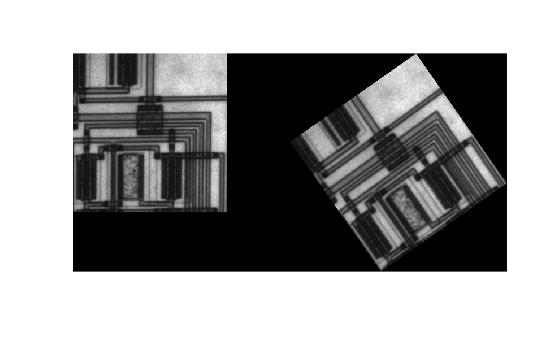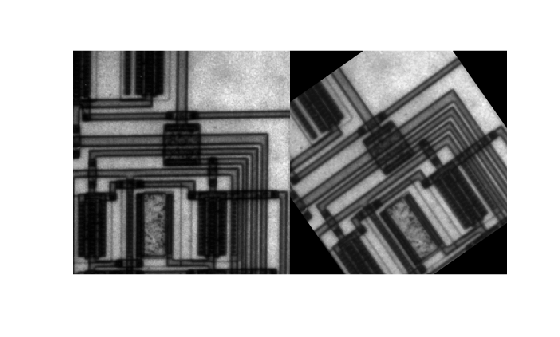# Rotate an Image

This example shows how to rotate an image and adjust the size of the resulting image.

When you rotate an image using the `imrotate` function, you specify the image to be rotated and the rotation angle, in degrees. If you specify a positive rotation angle, the image rotates counterclockwise; if you specify a negative rotation angle, the image rotates clockwise.

### Rotate an Image Counterclockwise

Read an image into the workspace.

`I = imread("circuit.tif");`

Rotate the image 35 degrees counterclockwise using bilinear interpolation.

`J = imrotate(I,35,"bilinear");`

Display the original image and the rotated image. By default, the output image is large enough to include the entire original image. Pixels that fall outside the boundaries of the original image are set to `0` and appear as a black background in the output image.

```figure imshowpair(I,J,"montage")```### Crop a Rotated Image

Rotate the original image again and specify that the rotated image be cropped to the same size as the original image.

`K = imrotate(I,35,"bilinear","crop");`

Display the original image and the new image.

```figure imshowpair(I,K,"montage")```